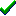# fg_3Daxisangleobject()Win32DirectXFastgraph/Light

Prototype

int fg_3Daxisangleobject (double x, double y, double z, int angle);

Function fg_3Daxisangleobject (ByVal x As Double, ByVal y As Double, ByVal z As Double, ByVal angle As Long) As Long

function fg_3Daxisangleobject (x, y, z : double; angle : integer) : integer;

Description

The fg_3Daxisangleobject() function rotates an object by the specified angle around an arbitrary axis through its origin.

Parameters

x is the x coordinate of a vector defining the axis of rotation.

y is the y coordinate of a vector defining the axis of rotation.

z is the z coordinate of a vector defining the axis of rotation.

angle is the rotation angle, expressed in tenths of degrees. If angle is positive, the object rotates clockwise. If angle is negative, the object rotates counterclockwise.

Return value

If successful, fg_3Daxisangleobject() returns zero. It returns -1 if the vector's magnitude is too close to zero.

Restrictions

none

See also

Contents
Fastgraph Home Page

copyright 2001 Ted Gruber Software, Inc.